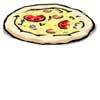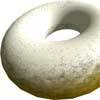#### You may also like### Pizza Portions

My friends and I love pizza. Can you help us share these pizzas equally?### Doughnut

How can you cut a doughnut into 8 equal pieces with only three cuts of a knife?### Red Balloons, Blue Balloons

Katie and Will have some balloons. Will's balloon burst at exactly the same size as Katie's at the beginning of a puff. How many puffs had Will done before his balloon burst?

# Pies

##### Age 7 to 11Challenge Level

Matthew and James recorded their answer as a fraction, 433 $\frac{1}{3}$ grams. Helena of Bebington and Christina, of Malborough Primary School, recorded their answers as decimal fractions. But each of these people used a combination of fractions and decimals to arrive at their solutions.

Matthew explained the procedure he used:

I got my answer of 433 $\frac{1}{3}$ grams by putting the problem into an equation. These are the steps I took to get the answer:

1. First I did the sum $200$g. + $125$g. which equalled $325$g.
2. Then I wrote it down like this: $325$g. + $\frac{1}{4}$ pie = pie
I then converted this to: $325$g. +$\frac{1}{4}$ pie = $\frac{4}{4}$ pie (or one whole pie).
3. Next, I took $\frac{1}{4}$ of a pie from each side leaving me with: $325$g. = $\frac{3}{4}$ pie.
4. I multiplied each side by $4$ which came out as: $1300$ = $3$ pies

But Matthew only wanted to find the weight of one pie, so he did this last important step:

5. I divided each side by $3$ and came to the final answer of:433 $\frac{1}{3}$ grams = $1$ pie."

Helena described her method this way:

$\frac{1}{4}$ pie = $325$g. divided by $3$, which is $108.3$g.
So, $4 \times \frac{1}{4}$ of a pie = $4 \times108.3$g. or $433.2$g.
$433.2$ grams is weight of a whole pie"